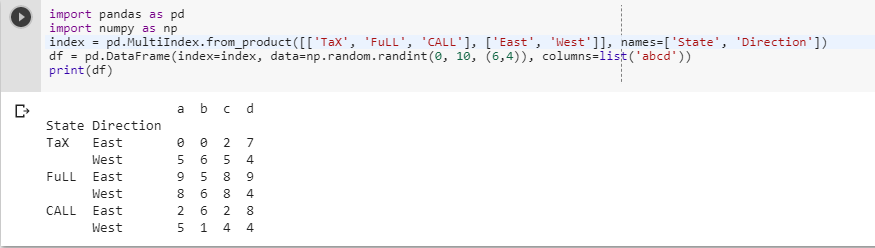2 views
in Python

This seems rather obvious, but I can't seem to figure out how to convert an index of data frame to a column?

For example:

df=

gi       ptt_loc

0 384444683  593

1 384444684  594

2 384444686  596

To,

df=

index1     gi       ptt_loc

0   0      384444683   593

1   1      384444684   594

2   2      384444686   596

by (106k points)

If you want to convert index of a pandas dataframe into a column you can use the following way:

df['index1'] = df.index

or

df.reset_index(level=0, inplace=True)

Another thing you can use to provide a bit more clarity is, look at a DataFrame with two levels in its index:

import pandas as pd

import numpy as np

index = pd.MultiIndex.from_product([['TaX', 'FuLL', 'CALL'], ['East', 'West']], names=['State', 'Direction'])

df = pd.DataFrame(index=index, data=np.random.randint(0, 10, (6,4)), columns=list('abcd'))

print(df)